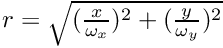BornAgain  1.19.0 Simulate and fit neutron and x-ray scattering at grazing incidence
Fourier-space distribution functions

## Classes

class  FTDistribution1DCauchy
Exponential IFTDistribution1D exp(-|omega*x|); its Fourier transform evaluate(q) is a Cauchy-Lorentzian starting at evaluate(0)=1. More...

class  FTDistribution1DCosine
IFTDistribution1D consisting of one cosine wave [1+cos(pi*x/omega) if |x|<omega, and 0 otherwise]; its Fourier transform evaluate(q) starts at evaluate(0)=1. More...

class  FTDistribution1DGate
Square gate IFTDistribution1D; its Fourier transform evaluate(q) is a sinc function starting at evaluate(0)=1. More...

class  FTDistribution1DGauss
Gaussian IFTDistribution1D; its Fourier transform evaluate(q) is a Gaussian starting at evaluate(0)=1. More...

class  FTDistribution1DTriangle
Triangle IFTDistribution1D [1-|x|/omega if |x|<omega, and 0 otherwise]; its Fourier transform evaluate(q) is a squared sinc function starting at evaluate(0)=1. More...

class  FTDistribution1DVoigt
IFTDistribution1D that provides a Fourier transform evaluate(q) in form of a pseudo-Voigt decay function eta*Gauss + (1-eta)*Cauchy, with both components starting at 1 for q=0. More...

class  FTDistribution2DCauchy
Two-dimensional Cauchy distribution in Fourier space; corresponds to a normalized exp(-r) in real space, with. More...

class  FTDistribution2DCone
Two-dimensional cone distribution in Fourier space; corresponds to 1-r if r<1 (and 0 otherwise) in real space with. More...

class  FTDistribution2DGate
Two-dimensional gate distribution in Fourier space; corresponds to normalized constant if r<1 (and 0 otherwise) in real space, with. More...

class  FTDistribution2DGauss
Two-dimensional Gauss distribution in Fourier space; corresponds to normalized exp(-r^2/2) in real space with. More...

class  FTDistribution2DVoigt
Two-dimensional Voigt distribution in Fourier space; corresponds to eta*Gauss + (1-eta)*Cauchy. More...